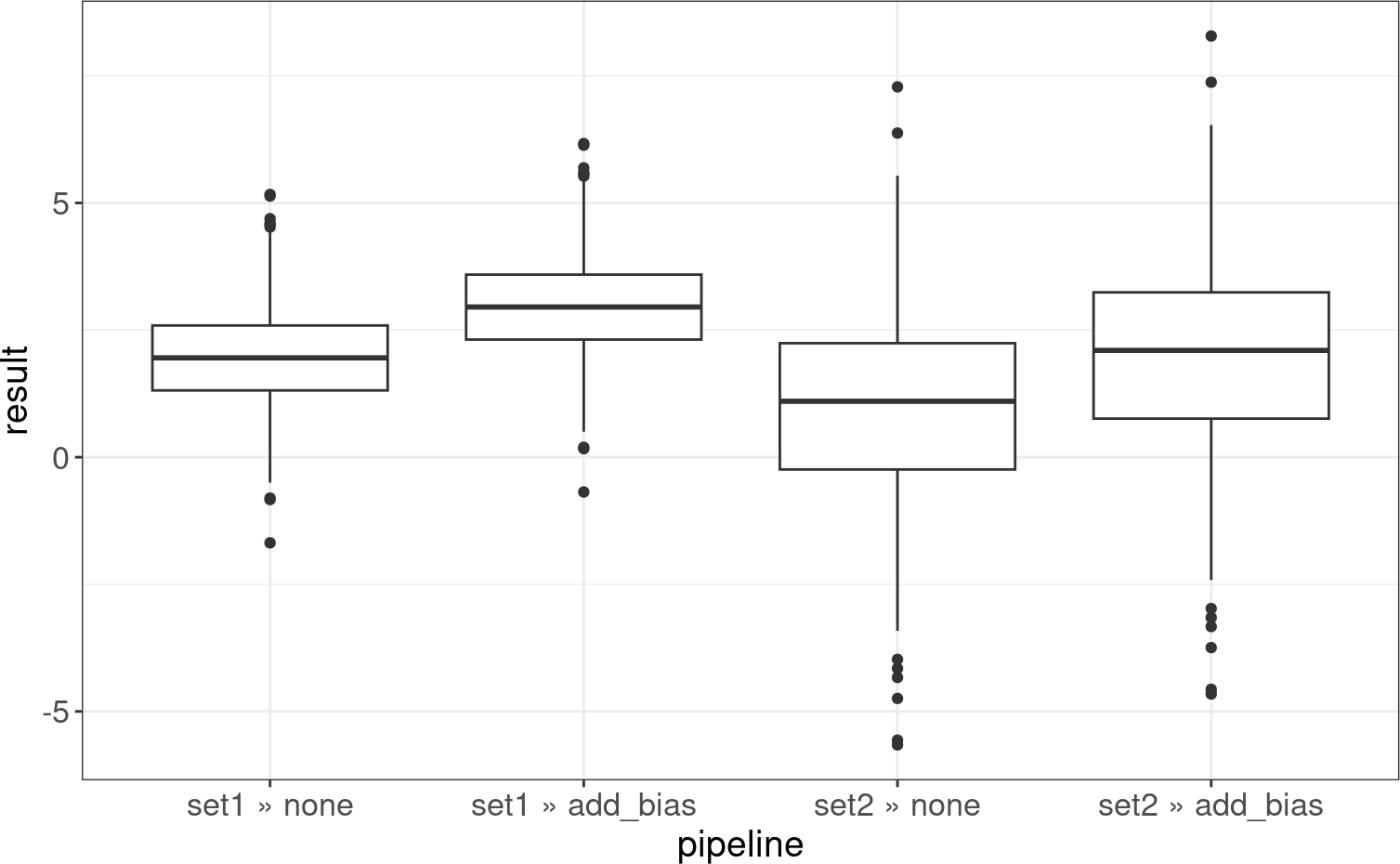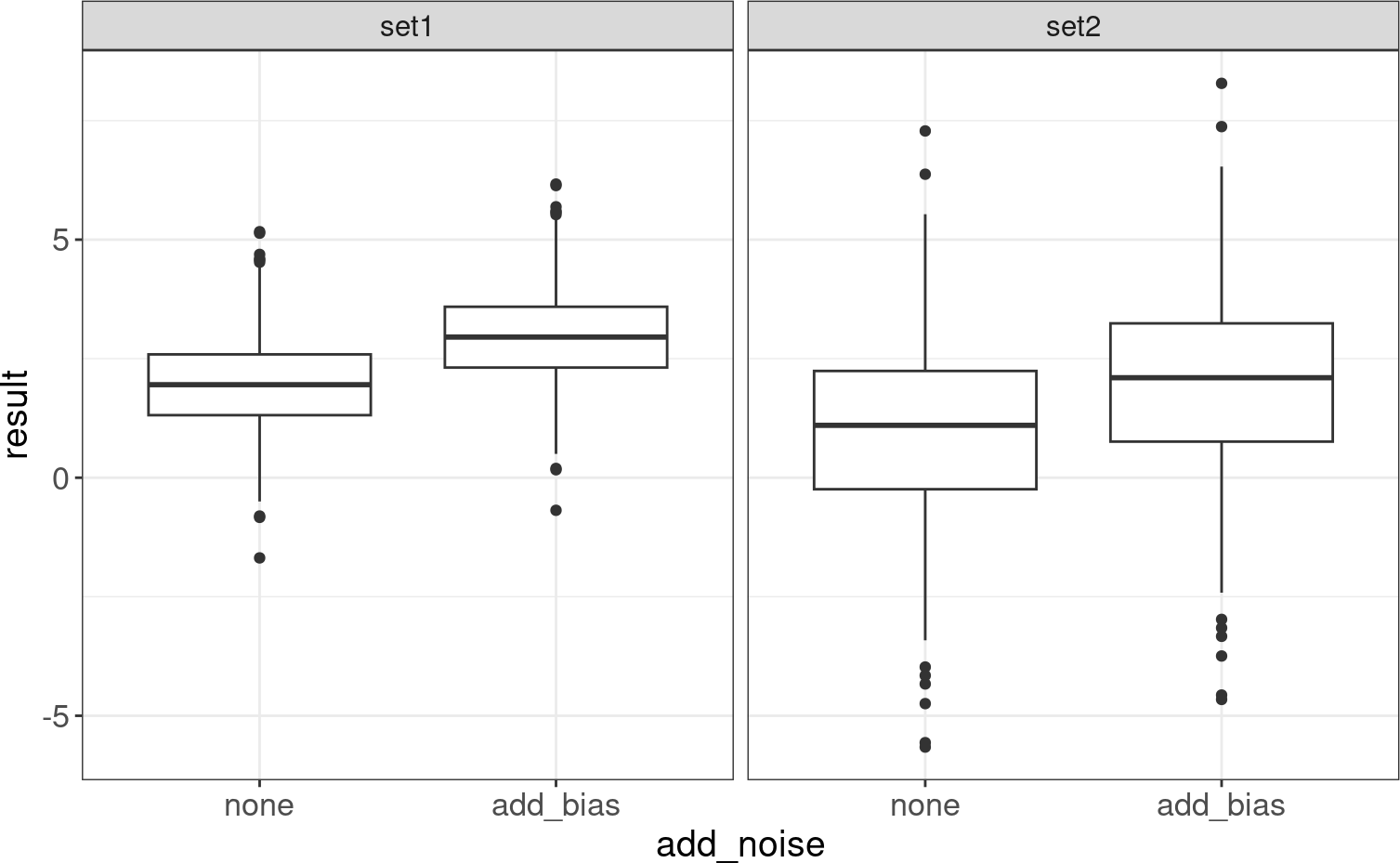# 1 Introduction

This vignette will introduce tidyverse patterns that are useful for making full use of the CellBench framework. CellBench was developed with tidyverse compatibility as a fundamental goal. purrr provides functional programming tools to manipulate the methods and lists produced in this framework. dplyr is very useful for working with the tibble-based structures produced by CellBench. Since the outputs are mostly in tibble structure, they are very easily visualised using ggplot2.

For detailed explanations of tidyverse packages please see resources at https://www.tidyverse.org/learn/, in particular R for Data Science.

For quick concise references of tidyverse features and functions I recommend all of the cheatsheets available at https://www.rstudio.com/resources/cheatsheets/.

# 2 Functional Programming with purrr

## 2.1 Methods as Function Objects

In CellBench we require methods to take in only a single argument. The idea is that all methods within a single pipeline step should take the same kind of input and produce the same type of output. In practice most methods have additional parameters that can be tuned, and we may use purrr::partial() to help pre-fill these parameters.

partial() takes a function, some variable values, and returns the function with the specified arguments pre-filled. This reduces the number of free parameters in your function.

We demonstrate a trivial application of partial():

library(CellBench)
library(purrr)

# function to raise number to a power
pow <- function(x, n) {
x^n
}

pow2 <- partial(pow, n = 2)
pow3 <- partial(pow, n = 3)

pow2(2)
##  4
pow3(2)
##  8

Here partial() allowed us to turn a two-parameter function into a single parameter function. Using partial() is good practice compared to the two alternatives:

1. Writing duplicate functions
2. Writing function wrappers

Consider writing duplicate functions

pow2 <- function(x) {
x^2
}

pow3 <- function(x) {
x^3
}

Now say you wanted to add an argument check stopifnot(is.numeric(x)), you would need to edit the code in two places. The chances for errors leading to inconsistencies increases dramatically with the number of duplications and changes required.

Consider writing function wrappers

pow <- function(x, n) {
x^n
}

pow2 <- function(x) {
pow(x, 2)
}

pow3 <- function(x) {
pow(x, 3)
}

The issue here is that these functions can contain much more in their bodies than the simple function call. When working in collaboration or sharing the code in general, it’s not immediately clear that the intention of the derived functions is only to pre-fill certain variables. Using partial() is concise and unambiguous in purpose.

• ?partial in the example section for more ways to use partial
• ?fn_arg_seq for the CellBench utility to construct a list of functions with varying parameter values

## 2.2 Function Composition

When functions have just one argument, they can easily be composed to create new functions. We use purrr::compose() for this, and it takes a series of functions as input. compose() will then return a function that applies the functions given to it in a right-to-left fashion, such that the right-most function is applied first and the left-most is applied last in succession.

# find the maximum absolute value
max_absolute <- compose(max, abs)

max_absolute(rnorm(100))
##  2.470413

This is useful for stitching together steps of a pipeline manually, for example if some methods require normalisation but some do not, then you may write wrappers as follows

method1 <- function(x) {
x <- normalise(x)
method_func1(x)
}

method2 <- function(x) {
method_func2(x)
}

method3 <- function(x) {
x <- normalise(x)
method_func3(x)
}

alternatively you could write

# identity simply returns its argument, useful here for code consistency
method1 <- compose(method_func1, normalise)
method2 <- compose(method_func2, identity)
method3 <- compose(method_func3, normalise)

which is more succinct and likely to be less error-prone. This is useful when two or more steps in a pipeline would only work in specific combinations, then these combinations can be fused together to form a single step in the desired combinations.

## 2.3 Mapping Over Lists

The majority of purrr’s functionality revolves around mapping functions over a list of inputs. This is a represented by the map() family of functions that usually take a list and a function as arguments, returning the result of applying the function to each element of the list.

map() is the primary function which performs basic mapping of lists and returns list. It functions almost identically to lapply, but is accompanied by a family of suffixed variants that are useful for various situations.

x <- list(1, 2, 3)

map(x, function(x) { x * 2 })
## []
##  2
##
## []
##  4
##
## []
##  6

One useful variant is map2() which takes two lists and applies a two-argument function to the first elements of both lists, second elements and so on. This can be used to pass additional variables into the function.

# list of random values from different distributions
x <- list(
rpois(100, lambda = 5),
rpois(100, lambda = 5),
rgamma(100, shape = 5),
rgamma(100, shape = 5)
)

y <- list(
"mean",
"median",
"mean",
"median"
)

# function that takes values and a mode argument
centrality <- function(x, mode = c("mean", "median")) {
mode <- match.arg(mode)

if (mode == "mean") {
mean = mean(x)
} else if (mode == "median") {
median = median(x)
}
}

# using map2 to apply function to two lists
map2(x, y, centrality)
## []
##  4.82
##
## []
##  5
##
## []
##  5.393148
##
## []
##  4.918361

# 3 Table Manipulation with dplyr

## 3.1 Operations on the Benchmark tibble

The fundamental benchmark_tbl() is derived from the tibble object which acts mostly indentical to a regular data.frame. Therefore it is compatible with the dplyr set of table manipulation functions.

library(dplyr)
# list of data
datasets <- list(
set1 = rnorm(500, mean = 2, sd = 1),
set2 = rnorm(500, mean = 1, sd = 2)
)

# list of functions
none = identity,
add_bias = function(x) { x + 1 }
)

class(res)
##  "benchmark_tbl" "tbl_df"        "tbl"           "data.frame"
res
## # A tibble: 4 × 3
##   <fct> <fct>     <list>
## 1 set1  none      <dbl >
## 2 set1  add_bias  <dbl >
## 3 set2  none      <dbl >
## 4 set2  add_bias  <dbl >

From our results we can filter the rows or manipulat the columns with regular dplyr operations.

# filtering rows to only data from set 1
res %>%
filter(data == "set1")
## # A tibble: 2 × 3
##   <fct> <fct>     <list>
## 1 set1  none      <dbl >
## 2 set1  add_bias  <dbl >
# filtering rows to only add_bias method
res %>%
filter(add_noise == "add_bias")
## # A tibble: 2 × 3
##   <fct> <fct>     <list>
## 1 set1  add_bias  <dbl >
## 2 set2  add_bias  <dbl >
# mutating data column to prepend "data" to data set names
res %>%
mutate(data = paste0("data", data))
## # A tibble: 4 × 3
##   <chr>    <fct>     <list>
## 1 dataset1 none      <dbl >
## 2 dataset1 add_bias  <dbl >
## 3 dataset2 none      <dbl >
## 4 dataset2 add_bias  <dbl >

## 3.2 Calculating multiple columns of metrics

We often want to plot two or more metrics against each other, for this purpose it is most useful to have each metric in its own column. The default CellBench model does not appear to support this, but it can be done quite easily using spread() from the tidyr package.

metric <- list(
mean = mean,
median = median
)

# simply applying the metrics results in a single column
res %>%
apply_methods(metric)
## # A tibble: 8 × 4
##   <fct> <fct>     <fct>   <dbl>
## 1 set1  none      mean    1.97
## 2 set1  none      median  1.95
## 3 set1  add_bias  mean    2.97
## 4 set1  add_bias  median  2.95
## 5 set2  none      mean    0.930
## 6 set2  none      median  1.10
## 7 set2  add_bias  mean    1.93
## 8 set2  add_bias  median  2.10
# spread metrics across columns
res %>%
apply_methods(metric) %>%
spread(metric, result)
## # A tibble: 4 × 4
##   <fct> <fct>     <dbl>  <dbl>
## 1 set1  none      1.97    1.95
## 2 set1  add_bias  2.97    2.95
## 3 set2  none      0.930   1.10
## 4 set2  add_bias  1.93    2.10

# 4 Plotting with ggplot2

## 4.1 Basic Plotting

Tibble results are easy to use with ggplot2. For a more extensive introduction to ggplot2 see R for Data Science: Chapter 3. Here we will plot results of our pipelines in a single plot, because the result column is a list-column, it needs to be unnested to produce a “flat” table, see ?tidyr::unnest for more explanation on unnesting. For convenience, we also use pipeline_collapse() from CellBench to concatenate the method names at each stop to produce a single character string representing a pipeline.

library(tidyr)
library(ggplot2)

# I prefer my own theme for ggplot2, following theme code is optional
theme_set(theme_bw() + theme(
plot.title = element_text(face = "plain", size = rel(20/12),
hjust = 1/2, margin = margin(t = 10, b = 20)),
axis.text = element_text(size = rel(14/12)),
strip.text.x = element_text(size = rel(16/12)),
axis.title = element_text(size = rel(16/12))
))

scale_colour_discrete <- function(...) scale_colour_brewer(..., palette="Set1")
scale_fill_discrete <- function(...) scale_fill_brewer(... , palette="Set1")
# pipeline collapse constructs a single string from the pipeline steps,
# unnest expands the list-column of results, transforming the result
# into a flat table.
collapsed_res <- pipeline_collapse(res) %>%
unnest()
## Warning: cols is now required when using unnest().
## ℹ Please use cols = c(result).
ggplot(collapsed_res, aes(x = pipeline, y = result)) +
geom_boxplot()## 4.2 Facetting

We can “facet” the above plot by sectioning off related graphics. This general idea covered in the facet section of the previously linked text. This also demonstrates the benefits of having the data in a tibble format.

# remember that we have to unnest the data before it's appropriate
# for plotting
ggplot(unnest(res), aes(x = add_noise, y = result)) +
geom_boxplot() +
facet_grid(~data)
## Warning: cols is now required when using unnest().
## ℹ Please use cols = c(result).